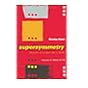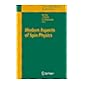Normal view

# Fundamentals of chemistry / David E. Goldberg.

Material type:TextLanguage: English Publisher: Dubuque, Iowa : Wm. C. Brown, c1994Description: xxx, 506 p. : col. ill. ; 27 cmISBN: 0697127990; 9780697127990DDC classification: 540 LOC classification: QD33 | .G64 1994Online resources: WorldCat details
Contents:
Table of contents Basic concepts; measurements; atoms and atomic masses; electronic configuration of the atom; chemical bonding; nomenclature; formula calculations; chemical reactions; stoichiometry; net ionic equations; molarity; gases; solids and liquids; solutions; oxidation numbers; reaction rates and chemical equilibrium; acid-base theory; organic chemistry; nuclear reactions. Appendix: scientific calculations; tables of symbols, abbreviations, prefixes and suffixes; table of basic mathematical equations; answers to practice problems; answers to end-of-chapter problems.
Summary: Summary: An introduction to the fundamentals of chemistry, intended for students who need help with mathematical manipulations, as well as reading and writing scientific research.
Tags from this library: No tags from this library for this title.Average rating: 0.0 (0 votes)
Item type Current location Collection Call number Copy number Status Date due Barcode Item holdsText
Reserve Section
Non-fiction 540 GOF (Browse shelf) C-1 Not For Loan 16446
Total holds: 0
##### Browsing EWU Library shelves, Shelving location: Reserve Section Close shelf browser539.725 KAS 2000 Supersymmetry : 539.725 MOD 2007 Modern aspects of spin physics / 540 CHE Essential chemistry / 540 GOF Fundamentals of chemistry / 540 KAG General chemistry / 540 SCC Chemistry in context : 540.20 ZUC Chemical principles /

Periodic table of the elements, and Table of the elements on endpapers.

Includes bibliographical references (p. xxvi-xxvii) and index.

Table of contents Basic concepts; measurements; atoms and atomic masses; electronic configuration of the atom; chemical bonding; nomenclature; formula calculations; chemical reactions; stoichiometry; net ionic equations; molarity; gases; solids and liquids; solutions; oxidation numbers; reaction rates and chemical equilibrium; acid-base theory; organic chemistry; nuclear reactions. Appendix: scientific calculations; tables of symbols, abbreviations, prefixes and suffixes; table of basic mathematical equations; answers to practice problems; answers to end-of-chapter problems.

Summary:
An introduction to the fundamentals of chemistry, intended for students who need help with mathematical manipulations, as well as reading and writing scientific research.

Pharmacy

There are no comments for this item.